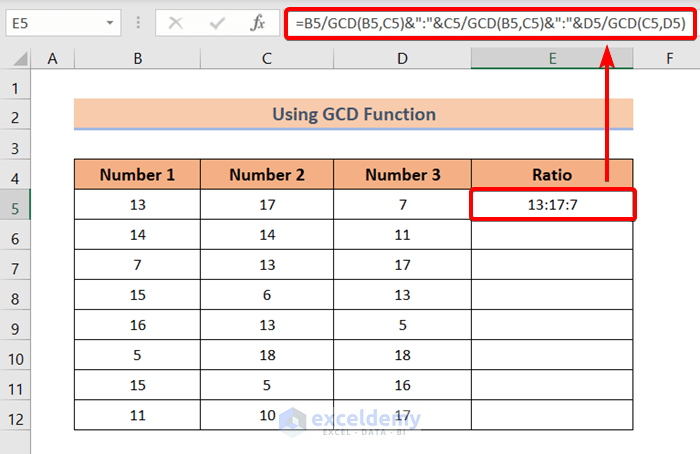# How to Calculate Ratio of 3 Numbers in Excel (3 Quick Methods)

Get FREE Advanced Excel Exercises with Solutions!

The calculating ratio of numbers can be very helpful in analyzing data by comparison. Excel doesn’t have any built-in feature or function that can be used to calculate the ratio of 3 numbers. However, we can use formulas to fill that void. In this article, I will discuss 3 formulas that you can use to calculate the ratio of 3 numbers in Excel with ease.

## How to Calculate Ratio of 3 Numbers in Excel: 3 Useful Methods

I’m going to use the following dataset which has four columns in total. I’ve used 3 columns named Number 1, Number 2, and Number 3. In these three columns, I’ve inserted some random numbers. In the fourth column called Ratio, I will use formulas to calculate the ratio of 3 numbers from Number 1, Number 2, and Number 3 columns.### 1. Using Basic Division to Calculate Ratio of 3 Numbers

We can calculate the ratio of 3 numbers using the basic division formula quite easily.

All you need to do is,

❶ Insert the following formula in cell E5.

`="1:"&C5/B5&":"&D5/B5`

❷ After that press ENTER.

Formula Breakdown

If we divide all 3 numbers by the first number, then we get the ratio of the 3 numbers with respect to the first number. The above formula is written based on this concept.

• B5, C5, D5 represent the Number 1, Number 2, and Number 3
• Dividing B5 with B5 always produces 1. Thus, I kept 1 as the default ratio of the Number 1.
• C5/B5 calculates the ratio of Number 2.
• D5/B5 calculates the ratio of Number 3.
• Finally, each ratio is separated by colons (:) from one another.❸ Drag the Fill Handle icon from cell E5 to E12.Finally, you will get the ratio of 3 numbers in the Ratio column.This formula displays up to 15 decimal places for the fraction ratios. Which you may not need at all.

In the next method, I have shown how to round off fraction ratio numbers.

Read More: How to Calculate Average Ratio in Excel

### 2. Calculating Ratio of 3 Numbers with TEXT Function

In this method, I will show you how to calculate the ratio of 3 numbers with the TEXT function. The TEXT function allows us to add formats to the numbers.

In the previous method, the fraction ratio numbers were having long trails of decimal places. To trim those trailing decimal places I’m using the TEXT function here. I will round off the fraction ratio number to 2 decimal places.

❶  At first, insert the following formula in cell E5.

`="1:"&TEXT(C5/B5,"0.00")&":"&TEXT(D5/B5,"0.00")`

❷ After that press ENTER.

Formula Breakdown

• B5, C5, D5 represent the Number 1, Number 2, and Number 3
• Dividing B5 with B5 always produces 1. Thus, I kept 1 as the default ratio of the Number 1.
• C5/B5 calculates the ratio of Number 2.
• D5/B5 calculates the ratio of Number 3.
• Each ratio is separated by colons (:) from one another.
• The TEXT function rounds off the fraction numbers up to 2 decimal places. This is specified by “0.00”.❸ Drag the Fill Handle icon from cell E5 to E12.Finally, you will get the ratio of 3 numbers in the Ratio column.### 3. Using GCD Function to Calculate Ratio of 3 Numbers

If you want to calculate the ratio of 3 numbers in terms of integer numbers, you can use the GCD function.

In the previous two methods, I have shown you calculate the ratio of 3 numbers by dividing each number by the first number.

But here I will calculate the ratio of 3 numbers by dividing each number by the Greatest Common Divisor (GCD) of the other two numbers.

For that,

❶ Insert the following formula in cell E5.

`=B5/GCD(B5,C5)&":"&C5/GCD(B5,C5)&":"&D5/GCD(C5,D5)`

❷ After that press ENTER.

Formula Breakdown

• B5, C5, D5 represent the Number 1, Number 2, and Number 3
• The GCD function returns the Greatest Common Divisor of two numbers.
• B5/GCD(B5,C5) returns the proportion of Number 1.
• C5/GCD(B5,C5) returns the proportion of Number 2.
• D5/GCD(C5,D5) returns the proportion of Number 3.
• Finally, each ratio is separated by colons (:) from one another.

###❸ Drag the Fill Handle icon from cell E5 to E12.Finally, you will get the ratio of 3 numbers in the Ratio column.Read More: How to Calculate Sortino Ratio in Excel

## Conclusion

To sum up, we have discussed 3 ways to calculate the ratio of 3 numbers in Excel. And don’t hesitate to ask any questions in the comment section below. We will try to respond to all the relevant queries ASAP.

## What is ExcelDemy?

ExcelDemy Learn Excel & Excel Solutions Center provides free Excel tutorials, free support , and premium Excel consultancy services for Excel and business users. Feel free to contact us with your Excel projects.Mrinmoy Roy

Hi! I'm Mrinmoy Roy. I'm an Excel and VBA content developer. I write blogs relating to Microsoft Excel on Exceldemy.com. I've completed my graduation in Electronics and Communication Engineering from Khulna University of Engineering & Technology. I've expertise in Excel functions, formulas, Pivot Table, Power Query, Visual Basic, etc. I write blogs to lessen people's hassles while working on Microsoft Excel.

We will be happy to hear your thoughtsAdvanced Excel Exercises with Solutions PDF